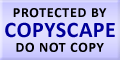Submit Assignment From Here
Name
:
Email
:
Phone
:
Country
:
Willing to pay US\$
:
Subject
:
Topic
:
Levels
:
:Hours
:

# Descriptive Statistics Homework Help

Descriptive Statistics means describing the main features of a data in a quantitative manner. It is summary of sample and observations. These summaries are summary statistics or easy to understand graphs. There is a big difference between descriptive statistics and inductive statistics. We can say that descriptive Statistics is used to shrink the sample size or in other words it can be used to summarize the sample. Hence, we can say that the basis of descriptive Statistics is not probability. When a data is presented in a conclusive manner the descriptive Statistics are also presented with the inductive statistics. To illustrate this we can say that, if we want to conduct a population survey there is basically table which has the sample size, demographic area, clinical characters like age, proportion of sex etc. The measures used for description of data are of central tendency. These measures include the mean, the median and the mode and measures of dispersion include standard deviation, kurtosis and skewness. For example runs scored in cricket is a descriptive Statistics that gives us a summary about the game or the player as a whole. This number is calculated as follows: Number of runs scored by a player/total number of runs scored by the team.US